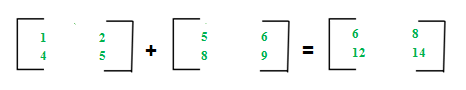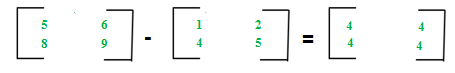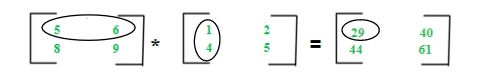GATE  >  Different Operations on Matrices

# Different Operations on Matrices Notes | Study Engineering Mathematics - GATE

## Document Description: Different Operations on Matrices for GATE 2022 is part of Engineering Mathematics preparation. The notes and questions for Different Operations on Matrices have been prepared according to the GATE exam syllabus. Information about Different Operations on Matrices covers topics like and Different Operations on Matrices Example, for GATE 2022 Exam. Find important definitions, questions, notes, meanings, examples, exercises and tests below for Different Operations on Matrices.

Introduction of Different Operations on Matrices in English is available as part of our Engineering Mathematics for GATE & Different Operations on Matrices in Hindi for Engineering Mathematics course. Download more important topics related with notes, lectures and mock test series for GATE Exam by signing up for free. GATE: Different Operations on Matrices Notes | Study Engineering Mathematics - GATE
 1 Crore+ students have signed up on EduRev. Have you?

The addition of two matrices Am * n and Bm * n gives a matrix Cm * n. The elements of C are sum of corresponding elements in A and B which can be shown as:The algorithm for addition of matrices can be written as:
for i in 1 to m
for j in 1 to n
cij = aij + bij
// C++ Program for matrix addition
#include <iostream>
using namespace std;
int main()
{
int n = 2, m = 2;
int a[n][m] = { { 2, 5 }, { 1, 7 } };
int b[n][m] = { { 3, 7 }, { 2, 9 } };
int c[n][m];
for (int i = 0; i < n; i++)
for (int j = 0; j < n; j++) {
c[i][j] = a[i][j] + b[i][j];
}
for (int i = 0; i < n; i++) {
for (int j = 0; j < n; j++)
cout << c[i][j] << " ";
cout << endl;
}
}

Output:
5 12
3 16

Time Complexity: O(n * m)

Auxiliary Space: O(n * m)

Key points:

• Addition of matrices is commutative which means A + B = B + A
• Addition of matrices is associative which means A + (B + C) = (A + B) + C
• The order of matrices A, B and A + B is always same
• If order of A and B is different, A + B can’t be computed
• The complexity of addition operation is O(m * n) where m * n is order of matrices
Matrices Subtraction

The subtraction of two matrices Am * n and Bm * n gives a matrix Cm * n. The elements of C are difference of corresponding elements in A and B which can be represented as:The algorithm for subtraction of matrices can be written as:
for i in 1 to m
for j in 1 to n
cij = aij-bij
// C++ Program for matrix substraction
#include <iostream>
using namespace std;
int main()
{
int n = 2, m = 2;
int a[n][m] = { { 2, 5 }, { 1, 7 } };
int b[n][m] = { { 3, 7 }, { 2, 9 } };
int c[n][m];
for (int i = 0; i < n; i++)
for (int j = 0; j < n; j++) {
c[i][j] = a[i][j] - b[i][j];
}
for (int i = 0; i < n; i++) {
for (int j = 0; j < n; j++)
cout << c[i][j] << " ";
cout << endl;
}
}

Output:
-1 -2
-1 -2

Key points:

• Subtraction of matrices is non-commutative which means A - B ≠ B - A
• Subtraction of matrices is non-associative which means A - (B - C) ≠ (A - B) - C
• The order of matrices A, B and A - B is always same
• If order of A and B is different, A - B can’t be computed
• The complexity of subtraction operation is O(m * n) where m * n is order of matrices

Matrices Multiplication

The multiplication of two matrices Am * n and Bn * p gives a matrix Cm * p. It means number of columns in A must be equal to number of rows in B to calculate C = A * B. To calculate element c11, multiply elements of 1st row of A with 1st column of B and add them (5 * 1 + 6 * 4) which can be shown as:The algorithm for multiplication of matrices A with order m*n and B with order n*p can be written as:
for i in 1 to m
for j in 1 to p
cij = 0
for k in 1 to n
cij + = aik * bkj
// C++ Program for matrix Multiplication
#include <iostream>
using namespace std;
int main()
{
int n = 2, m = 2;
int a[n][m] = { { 2, 5 }, { 1, 7 } };
int b[n][m] = { { 3, 7 }, { 2, 9 } };
int c[n][m];
int i, j, k;
for (i = 0; i < n; i++)
{
for (j = 0; j < n; j++)
{
c[i][j] = 0;
for (k = 0; k < n; k++)
c[i][j] += a[i][k] * b[k][j];
}
}
for (int i = 0; i < n; i++)
{
for (int j = 0; j < n; j++)
cout << c[i][j] << " ";
cout << endl;
}
}

Output:
16 59
17 70

Key points:

• Multiplication of matrices is non-commutative which means A * B ≠ B * A
• Multiplication of matrices is associative which means A * (B * C) = (A * B) * C
• For computing A * B, the number of columns in A must be equal to number of rows in B
• Existence of A * B does not imply existence of B * A
• The complexity of multiplication operation (A * B) is O(m * n * p) where m*n and n*p are order of A and B respectively
• The order of matrix C computed as A * B is m * p where m * n and n * p are order of A and B respectively.
The document Different Operations on Matrices Notes | Study Engineering Mathematics - GATE is a part of the GATE Course Engineering Mathematics.
All you need of GATE at this link: GATE

## Engineering Mathematics

45 videos|55 docs|21 tests
 Use Code STAYHOME200 and get INR 200 additional OFF

## Engineering Mathematics

45 videos|55 docs|21 tests

Track your progress, build streaks, highlight & save important lessons and more!

,

,

,

,

,

,

,

,

,

,

,

,

,

,

,

,

,

,

,

,

,

;